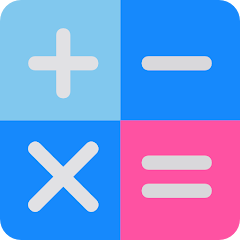# Mathematics Basic Concepts

10K+Everyone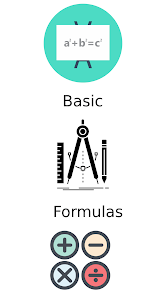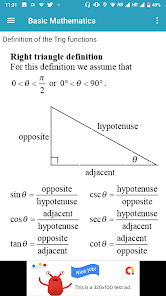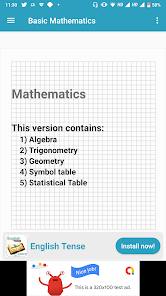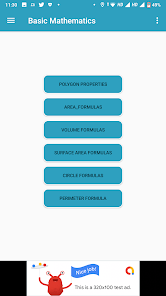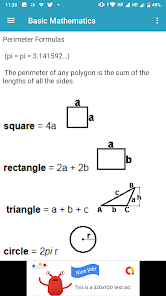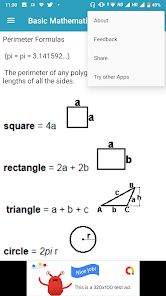Basic mathematics, pre-algebra, geometry, statistics, and algebra skills are what this App will teach you. It is designed for anyone who needs a basic to advanced understanding of mathematics concepts and operations.

Learn Basic Math

Basic math is nothing but a simple or basic concept related to mathematics. Generally, counting, addition, subtraction, multiplication, and division are called the basic math operation.

Basic Maths application is a very useful app for beginners to the maths.
Develop your basic math skills through fun and intuitive games makes learning math fun right from the beginning.

Why this app:
# Easy to use
# Fast
# Attractive

The app contains all the necessary formulas to help to solve from basics to advance problems. UI designed such that user will not experience any difficulty while working with the app, Navigation drawer is used in the app for a better user experience.

When you learn these mathematical tricks, you'll be able to show off your skills to friends and prove to them that you have a talent for mathematics. New skills you can use in the store, at school, at college, at work - wherever thanks to quick calculation skills can save a lot of precious time.

How do you start learning math?

You need to start in a logical and simple manner. Take some time to brush up on your high school mathematics. Then decide which branch of mathematics you want to pursue. If you have time, you can try enrolling in a college or a maths school

you'll find formulas very simply explained in the app with necessary figures that will help you to understand very easily.

Basic Mathematics Concepts is an easy-to-use app for students who are learning Mathematics as beginners. This app serves as an easy reference tool for some basic Mathematical formulas.

Learning math can be a daunting task when you do not have the right tools or the right materials, especially if you grew up believing that math is hard and that you will never understand it.

Learn Basic Math

Basic math is nothing but a simple or basic concept related to mathematics. Generally, counting, addition, subtraction, multiplication, and division are called basic math operations. The other mathematical concept is built on top of the above 4 operations. These concepts along with the different types of numbers, factors, lcm, and GCF make students ready for learning fractions.

For good learning of Basic Mathematics Courses, it is important to have easy access to the best Basic Mathematics Courses at any time.

On the following topics are obviously present in our application.

Preliminaries, Whole Numbers, Decimals, Fractions, Percents, Integers and Rationals, Powers Exponents and Roots, Powers of Ten and Scientific Notation, - Measurements, - Graphs, - Probabilities and Statistics, - Number Series
- Variables Algebraic Expressions and Simple Equations, - Word Problems
- Translating Expressions, - Equations

The app contains all the necessary formulas that help to solve from basics to advance problems.

App basically contains these sections of formulas

1.Algebra Formulas.
2.Trigonometry Formulas.
3.Geometry Formulas.
4.Statistical Table.
5.Symbols.

all Class Maths NCERT Solutions app is specially designed for the CBSE class students to help them prepare for their exams.

in this App - Mathematics Book And Its Solution Are Added initially there is NO NEED OF INTERNET Connection. And For Other Resource Need Only First time for file Download.

Solution

Sets
Relations And Functions
Trigonometric Functions
Principle Of Mathematical Induction
Linear Inequalities
Permutations And Combinations
Binomial Theorem
Sequence And Series
Straight Lines
Conic Sections
Introduction To Three Dimensional Geometry
Limits And Derivatives
Mathematical Reasoning
Statistics
Probability
Updated on
May 8, 2021

## Data safety